# Angular Momentum Conservation of Angular momentum Angular Momentum

• Slides: 9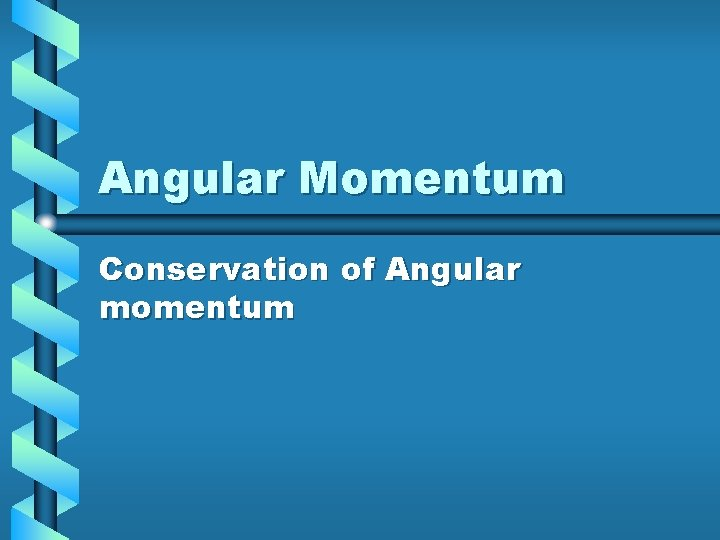Angular Momentum Conservation of Angular momentumAngular Momentum • L = r x p = r x mv • Magnitude L = rpsinθ = rmvsinθ • Derivative of angular momentum • d. L/dt = (dr/dt x mv) +(r x m dv/dt) = (v x mv) + (r x ma) = r x F = torque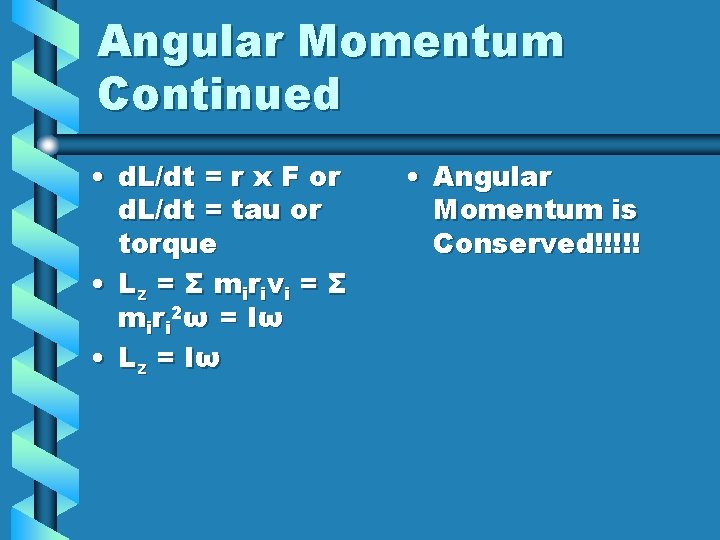Angular Momentum Continued • d. L/dt = r x F or d. L/dt = tau or torque • L z = Σ m ir iv i = Σ miri 2ω = Iω • Lz = Iω • Angular Momentum is Conserved!!!!!Problem • A string of negligible weight is wrapped around a pulley of mass M and radius R and tied to a mass m. The mass is released from rest and it drops a distance h to the floor. Use energy principles to determine the speed of the mass when it hits the floor. Determine the speed, tension and angular acceleration of the pulley.Solution • • KE 1 + U 1 = KE 2 + U 2 mgh = ½ mv 2 + 0 + ½ Iω2 I = ½ MR 2 , v = Rω mgh = ½ mv 2 +1/2(1/2 MR 2)(v/R)2 v 2 = 4 mgh/(2 m + M) v= √ 4 mgh/(2 m + M) TR = ½ MR 2α α = 2 T/MR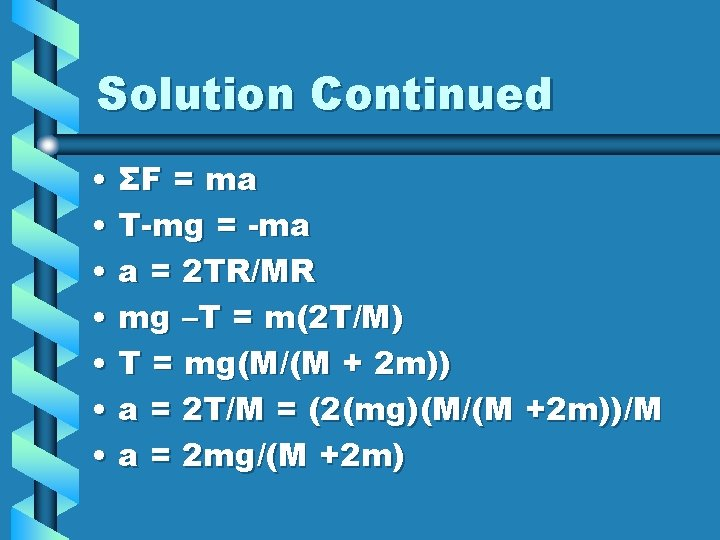Solution Continued • ΣF = ma • T-mg = -ma • a = 2 TR/MR • mg –T = m(2 T/M) • T = mg(M/(M + 2 m)) • a = 2 T/M = (2(mg)(M/(M +2 m))/M • a = 2 mg/(M +2 m)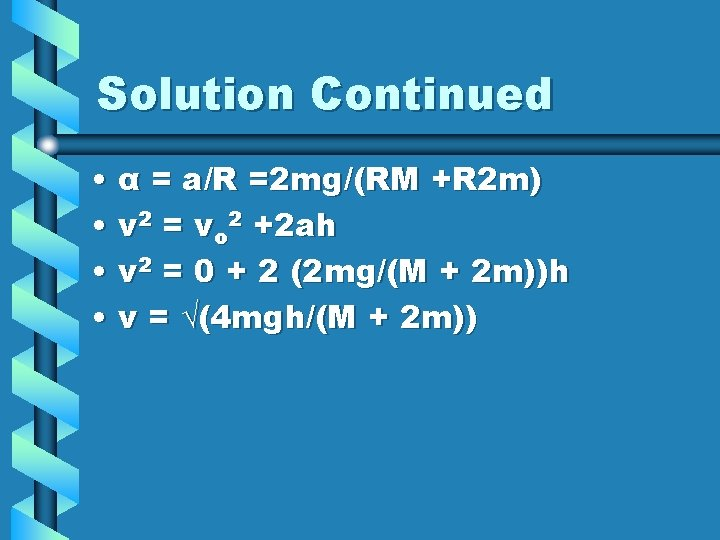Solution Continued • α = a/R =2 mg/(RM +R 2 m) • v 2 = vo 2 +2 ah • v 2 = 0 + 2 (2 mg/(M + 2 m))h • v = √(4 mgh/(M + 2 m))Problem 2 • A disk is mounted with its axis vertical. It has a radius R and a mass M. It is initially at rest. A bullet of mass m and a velocity v is fired horizontally and tangential to the disk. It lodges in the perimeter of the disk. What angular velocity will the disk acquire?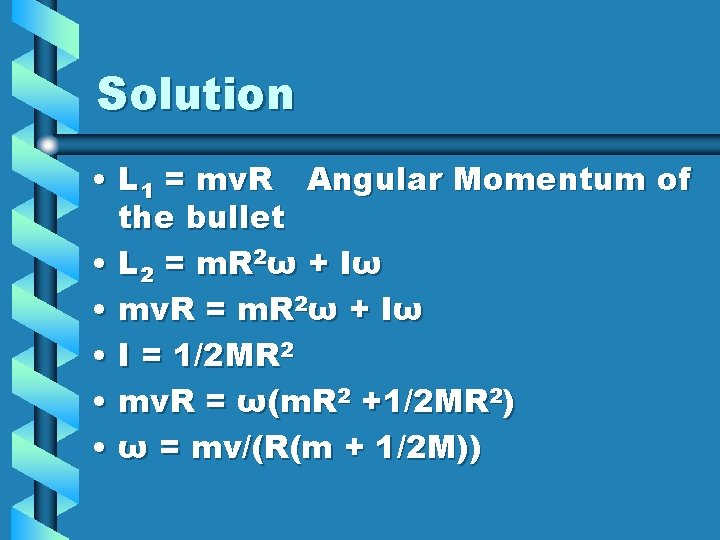Solution • L 1 = mv. R Angular Momentum of the bullet • L 2 = m. R 2ω + Iω • mv. R = m. R 2ω + Iω • I = 1/2 MR 2 • mv. R = ω(m. R 2 +1/2 MR 2) • ω = mv/(R(m + 1/2 M))